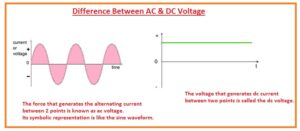Hello, friends, I hope you all are doing great. In today’s tutorial, we will discuss the Difference Between AC & DC Voltage. The basic difference among the AC voltage and DC voltage is the wave of ac voltage changes polarity after a certain time interval while dc voltage has constant polarity throughout the given time.

In today’s post, we will have a detailed look at both ac and dc voltages with the detailed compare them to find their differences according to their related parameters. So let’s get started with the Difference Between AC & DC Voltage.

#### Difference Between AC & DC Voltage

AC Voltage

• The force that generates the alternating current between 2 points is known as ac voltage.
• Its symbolic representation is like the sine waveform.
• AC voltage is generated through the ac generators like a synchronous generator and induction generators.
• The efficiency of the ac voltage is large.
• Its passive element is impedance.
• This voltage has some amplitude.
• AC volts are produced through ac generator like a synchronous generator.
• Ac volts transmission through longer distance very easily.
• It can be changed from one level to another through the use of a transformer
• Normally three-wire system used to ac voltage transmission
• Star and delta configuration used for transmission.
• The Star transmission system has four wires one phase and three neutral and the delta has three.
• The frequency value of ac voltage is different for different countries. Some countries use fifty-hertz frequency and some countries use sixty-hertz frequency.
• The polarity of ac voltage varies after a certain time interval.
• The direction of ac voltage does not remain constant.
• It can be transformed with the use of an inverter.
• AC voltage is used for the transmission of power.
• There is also the existence of phase and neutral for ac voltages.
• The benefit offered by the ac voltage is that it can find very easily.
• The value of the power factor for AC voltage is lies between zero and one.• Rectifier used for ac to dc conversion

DC Voltage

• The voltage that generates dc current between two points is called the dc voltage.
• Its symbolic representation is a straight line.
• It used to transmit through two-wire one phase and one neutral.
• Its generation is difficult than ac.
• Its transmission is costly.
• Can not be change value through the tranformer.
• Ac to dc conversion is done through a rectifier
• Its frequency is zero.
• The power factor value for dc voltage is zero.
• Its polarity does not vary.
• Its direction of propagation also not varies.
• It can be obtained from the dc battery.
• It provides less efficiency.
• Its passive parameter is resistance.
• There are no amplitude exits for dc voltage.
• The benefits offered by the dc voltage is that it can be easily amplified.That is a detailed post about the difference between ac and dc voltage. If you have any further query ask in comments. Thanks for reading. Have a good day.